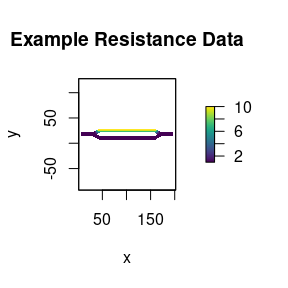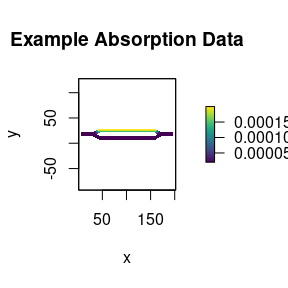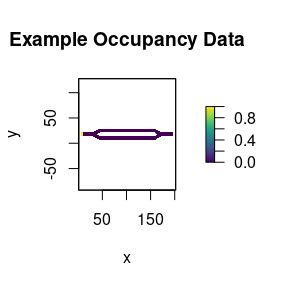# Overview

## Data

### Required Data

In order to use this package, two different sets of landscape data are required: resistance and absorption. There are certain requirements for these:

• They must be 2-dimensional matrices or RasterLayer objects. They have to be the same type.
• They must have the same dimensions (number of rows and columns).
• NA data is allowed in the cells, but must match between the sets of data. I.e., if cell [3, 6] of the resistance data has a NA value, then cell [3, 6] of the absorption data must also have a NA value, and vice versa.

If using RasterLayer objects, then additional conditions must be met:

• Both sets of data must have the same coordinate extents.
• Both sets of data must use the same coordinate reference system (CRS).

### Optional Data

The use of landscape fidelity data is optional. By default, the package treats all cells in the landscape data the same and uses a value of 0 for fidelity. If custom data is desired, then it must meet all of the same requirements listed above for the resistance and absorption landscape data.

### Built-in Example Data

The package includes built-in example data. Some of this data was used to create the figures in the SAMC paper, and is used in this tutorial. They are:

• ex_res_data: A matrix with landscape resistance data.
• ex_abs_data: A matrix with landscape absorption (mortality) data.
• ex_occ_data: A matrix with landscape occupancy data.
str(samc::ex_res_data)
#>  num [1:34, 1:202] NA NA NA NA NA NA NA NA NA NA ...
str(samc::ex_abs_data)
#>  num [1:34, 1:202] NA NA NA NA NA NA NA NA NA NA ...
str(samc::ex_occ_data)
#>  num [1:34, 1:202] NA NA NA NA NA NA NA NA NA NA ...

plot(raster(samc::ex_res_data, xmn = 1, xmx = ncol(samc::ex_res_data), ymn = 1, ymx = nrow(samc::ex_res_data)),
main = "Example Resistance Data", xlab = "x", ylab = "y", col = viridis(256))

plot(raster(samc::ex_abs_data, xmn = 1, xmx = ncol(samc::ex_abs_data), ymn = 1, ymx = nrow(samc::ex_abs_data)),
main = "Example Absorption Data", xlab = "x", ylab = "y", col = viridis(256))

plot(raster(samc::ex_occ_data, xmn = 1, xmx = ncol(samc::ex_occ_data), ymn = 1, ymx = nrow(samc::ex_occ_data)),
main = "Example Occupancy Data", xlab = "x", ylab = "y", col = viridis(256))## The samc-class

The samc-class is used to manage the transition matrix and information about your landscape data to help ensure that the calculations used by the rest of the package are used correctly. Creating an samc-class object is the mandatory first step in the package, and is created using the samc() utility function. The samc() function has several parameters. Some of these are mandatory, some are only mandatory in certain situations, and some are optional. The overall function signature is as follows:

samc(resistance, absorption, fidelity, latlon, tr_fun, p_mat, ...)

An explanation of the arguments:

• resistance and absorption are always mandatory, and must meet the data requirements in the Data section above.
• fidelity is optional. If included, it must meet the data requirements outlined in the Data section above.
• latlon is mandatory when the input data is in a RasterLayer object. It should be set to either TRUE or FALSE.
• tr_fun is always mandatory. It is used to create the transition matrix.
• directions is an optional parameter. It can be used to set how adjacent cells are connected.
• override is an optional parameter. It is used to control whether or not memory intensive functions can be run on the data. By default it is set to FALSE to prevent users from accidentally running these functions, which can potentially crash R if the computer does not have enough memory. The function documentation provides specific details for which calculations need the override, but in general it will rarely be useful for users and should be left off.

The p_mat parameter provides an alternative method for creating a samc-class directly from a preconstructed P matrix. See the samc() documentation for more details.

## Utility Functions

In addition to the extremely important samc() function, the package has other utility functions that users might find helpful:

• The check() function is used to check that input landscape data meets the data requirements outlined above. It can be used to compare two RasterLayer objects, two matrix objects, or check either a RasterLayer or a matrix against an already created samc-class object.
• The map() function is used to simplify mapping vector data back into the landscape and return it as a RasterLayer. This is provided because R handles matrices and raster layers somewhat differently when reading and writing vector data, which can cause users to map the data incorrectly if they aren’t careful. It also handles mapping to landscapes with NA values, another potential source of error.
• The locate() function is used to get cell numbers for use as origin and dest values in various analytical function arguments. This function should be used instead of cellFromXY() in the raster package because cellFromXY() cell numbers do not necessarily correspond to cell numbers in the samc package (the samc package does not assign cell numbers to NA cells, whereas the raster package does). The locate() function can be used to return a RasterLayer with the cell numbers encoded as cell values by simply excluding the xy argument.
• The pairwise() function is provided to easily and efficiently run specific metrics for all the pairwise combinations of start and end locations.

## Analytical Functions

The package implements functions for the formulas provided in Table 1 of Fletcher et al. (2019). Many of the formulas are related conceptually, and are grouped together into single functions with multiple parameter signatures to reduce the number of unique function names needed. Note that the descriptions assume $$\psi$$ contains probability of occurrence. If $$\psi$$ instead contains the number of individuals, then the metrics with $$\psi$$ will return the number of expected individuals rather than a probability.

Function Equation Description
cond_passage() $$\tilde{t} = \tilde{B}_j^{-1}\tilde{F}\tilde{B}_j{\cdot}1$$ Mean first conditional passage time
dispersal() $$\tilde{D}_{jt}=({\sum}_{n=0}^{t-1}\tilde{Q}^n)\tilde{q}_j$$ Probability of an individual visiting a location, if starting at any other location, before or at time t
$$\psi^T\tilde{D}_{jt}$$ Probability of an individual visiting a location, before or at time t, regardless of initial location
$$D=(F-I)diag(F)^{-1}$$ Probability of an individual visiting a location
$$\psi^TD$$ Probability of an individual visiting a location, regardless of initial location
distribution() $$Q^t$$ Probability of an individual being at a location at time t
$$\psi^TQ^t$$ Probability of an individual being at a location at time t, regardless of initial location
mortality() $$\tilde{B}_t = (\sum_{n=0}^{t-1} Q^n) \tilde{R}$$ Probability of an individual experiencing mortality at a location before or at time t
$$\psi^T \tilde{B}_t$$ Probability of an individual experiencing mortality at a location, before or at time t, regardless of initial location
$$B = F \tilde{R}$$ Probability of an individual experiencing mortality at a location
$$\psi^T B$$ Probability of an individual experiencing mortality at a location, regardless of initial location
survival() $$z=(I-Q)^{-1}{\cdot}1=F{\cdot}1$$ Expected life expectancy of an individual
$${\psi}^Tz$$ Overall life expectancy, regardless of initial location
visitation() $$F = (I-Q)^{-1}$$ Expected number of times an individual visits a location

Depending on the combination of inputs used, a function might return a single value, a vector, or a matrix. In some cases, the calculations will not be practical with sufficiently large landscape datasets due to memory and other performance constraints. To work around this, many equations have multiple associated function signatures that allow users to calculate individual portions of the result rather than the entire result. This opens up multiple optimizations that makes calculating many of the metrics more practical. More specific details about performance considerations can be found in the Performance vignette.